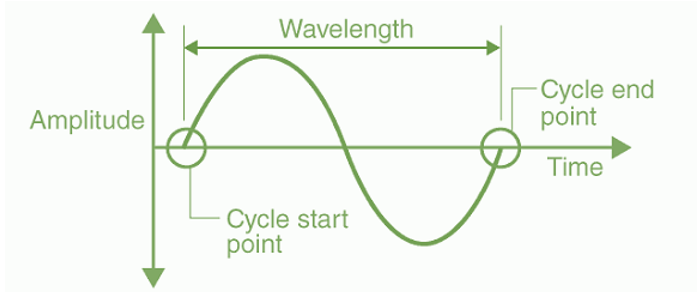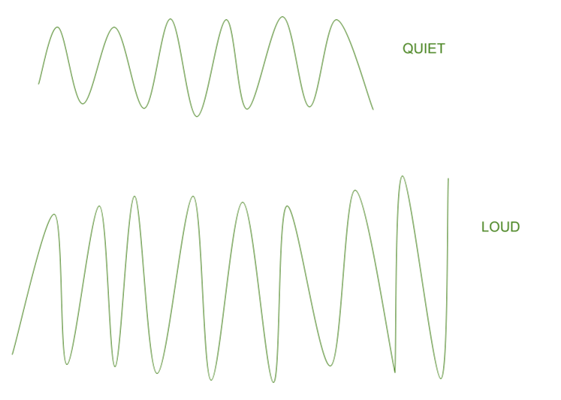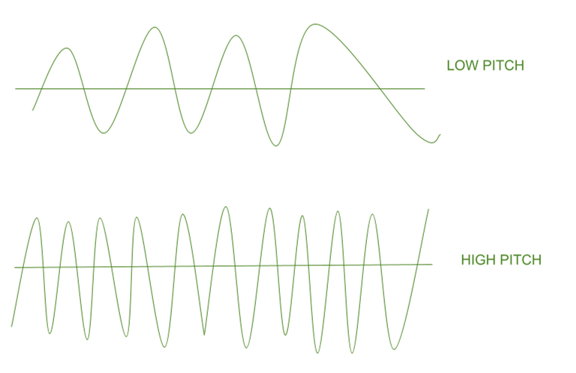GFG App
Open AppBrowser
Continue

One comes across various forms of sound in its daily lives, and everyone is well-equipped with a system to recognize various types of differences in the sound pattern. When a mother speaks to her child, her accent is distinct from that of the child. And all these variations are due to a mechanism called pitch and loudness of sound which always differentiates a person’s voice from another one. That’s why your voice is so unique in your whole class, family, and locality. However, a sound that closely resembles another or imitation also comes under this phenomenon. Let’s look at the loudness of sound, the pitch of the sound, and the differences between the two.

Before, discussing the concept of loudness and pitch of the sound let’s first briefly learn about the basic characteristics of sound. This would be of great help in understanding the loudness and pitch of the sound.

### Characteristics of Sound

One can hear all kinds of sounds in everyday life, like crying, yelling, and laughter and this isn’t limited to people. Animals make sounds as well, but they are not the same as human voices. Is the sound of a drum similar to that of a flute? So, what’s the difference between the two? Let’s look at some fundamental properties of sound to understand this.

There are main four characteristics of sound waves, explained briefly below as:

• Amplitude: The amount of energy in an electromagnetic wave is referred to as the amplitude of light. The maximum vertical displacement of the wave from its mean point is referred to as amplitude. The higher the energy, the greater the amplitude. The S.I unit of measurement of amplitude is meters (m) though sometimes it is also measured in centimeters.Amplitude and Wavelength

• Wavelength: The wavelength is the shortest distance over which a sound wave will repeat itself. In other words, the duration of a single full-wave is called the wavelength. A Greek letter is used to represent it, λ (lambda). The meter is the SI unit for measuring wavelength (m).
• Frequency: The time taken to create a single full-wave, cycle, or cycle is referred to as the wave’s time period. One maximum pulse of the vibrating body now produces one total wave. As a result, the time it takes to complete one vibration is referred to as the time period. The letter T stands for it. The second (s) is the unit of the time period.• Time-period: The frequency of a wave is defined as the number of full waves or cycles generated in one second. The number of waves per second is called frequency since one total wave is generated by one maximum pulse of the vibrating body. Hertz, or Hz, is the SI unit of frequency. The frequency of a vibrating body emitting one wave per second is 1 hertz. In other words, 1 Hz equals 1 pulse per second.

### Loudness of the SoundThe amount of energy exerted in producing a sound that can be measured by the reaching ear is called the loudness of a sound. It can also be termed as a response by the ear to the sound. The loudness of sound depends on its amplitude. If the amplitude of the vibration is large, the sound produced will also be loud. Whereas when the amplitude is small, the sound produced will also be feeble. The following are the important points related to the loudness of the sound:

• The loudness of sound is the measure of sound energy reaching the ear per second.
• The loudness of sound is directly proportional to the square of the amplitude of the vibration. That is,

Loudness ∝ (Amplitude)2

e.g.: If the amplitude becomes twice, the loudness will be 4 times.

• The property of loudness is the ability to differentiate between two sounds of the same frequency and pitch. The size and amplitude of sound waves determine the sound’s intensity.
• Loudness is expressed by a unit called decibel (dB). Above 80 dB the sound becomes noise that’s why babies are more sensitive to loud noises than adults.
• The table below provides a differentiated comparison over the loudness of a sound coming from various sources.

### Pitch of the SoundPitch defines the quality of a sound by determining its frequency scale. It is also known as shrillness. If the frequency of vibration is higher it means the sound is more shrill. But if the sound is of low frequency we will that the sound has a low pitch. For instance, it can be noticed that a woman’s voice is of a high pitch than the man’s voice. The following are the important points related to the pitch of the sound:

• This is determined by the magnitude of the waves’ vibrations.
• The sound is shrill and has a sharp pitch if the frequency of vibration is greater.
• When a sound is said to have a lower pitch, it means it vibrates at a lower frequency. A bird makes a high-pitched sound, while a lion’s scream is low-pitched.
• Human ears can hear sounds from 20 Hz to 20 kHz.
• Because of the differences in pitch, various species and objects produce different sounds:

### Sample Problems

Problem 1: If the amplitude of a wave is doubled, what will be the effect on its loudness?

Solution:

Since, it is known that, Loudness α (Amplitude)2.

Therefore, if amplitude is doubled then the loudness becomes 4 times.

Problem 2: What are the characteristics of musical sound?

Solution:

The three characteristics of a musical sound are:

Loudness, Pitch and Quality.

Problem 3: Why is the loudness of sound heard by a plucked wire increased when mounted on a soundboard?

Solution:

When a wire mounted on a sound board is plucked, the surface area of vibrating air increases and sends greater amount of energy. So the amplitude of vibration is large and louder is the sound heard.

Problem 4: State three factors on which loudness of sound heard by a listener depends?

Solution:

The various factors on which loudness of sound heard by a listener depends are:

1. Amplitude of wave,
2. Distance of source of sound,
3. Surface area of vibrating body,
4. Sensitivity of listener and
5. Intensity of sound.

Problem 5: Name and define the characteristic which enables one to distinguish two sounds of the same loudness, but of different frequencies, given by the same instrument.

Solution:

The characteristic which enables one to distinguish two sounds of same loudness, but of different frequencies, given by the same instrument is pitch. The sensation of a frequency is commonly referred to as the pitch of a sound. A high pitch sound corresponds to a high frequency and a low pitch sound refers to a low frequency sound wave.

My Personal Notes arrow_drop_up